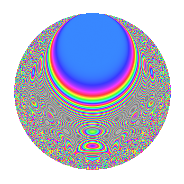Properties

 Label 6031.2.a.bLevel 6031 Weight 2 Character orbit 6031.a Self dual Yes Analytic conductor 48.158 Analytic rank 1 Dimension 109 CM No

Related objects

Newspace parameters

 Level: $$N$$ = $$6031 = 37 \cdot 163$$ Weight: $$k$$ = $$2$$ Character orbit: $$[\chi]$$ = 6031.a (trivial)

Newform invariants

 Self dual: Yes Analytic conductor: $$48.157777459$$ Analytic rank: $$1$$ Dimension: $$109$$ Fricke sign: $$1$$ Sato-Tate group: $\mathrm{SU}(2)$

$q$-expansion

The dimension is sufficiently large that we do not compute an algebraic $$q$$-expansion, but we have computed the trace expansion.

 $$\operatorname{Tr}(f)(q) =$$ $$109q$$ $$\mathstrut -\mathstrut 11q^{2}$$ $$\mathstrut -\mathstrut 14q^{3}$$ $$\mathstrut +\mathstrut 99q^{4}$$ $$\mathstrut -\mathstrut 28q^{5}$$ $$\mathstrut -\mathstrut 14q^{6}$$ $$\mathstrut -\mathstrut 16q^{7}$$ $$\mathstrut -\mathstrut 27q^{8}$$ $$\mathstrut +\mathstrut 65q^{9}$$ $$\mathstrut +\mathstrut O(q^{10})$$ $$\operatorname{Tr}(f)(q) =$$ $$109q$$ $$\mathstrut -\mathstrut 11q^{2}$$ $$\mathstrut -\mathstrut 14q^{3}$$ $$\mathstrut +\mathstrut 99q^{4}$$ $$\mathstrut -\mathstrut 28q^{5}$$ $$\mathstrut -\mathstrut 14q^{6}$$ $$\mathstrut -\mathstrut 16q^{7}$$ $$\mathstrut -\mathstrut 27q^{8}$$ $$\mathstrut +\mathstrut 65q^{9}$$ $$\mathstrut -\mathstrut 21q^{10}$$ $$\mathstrut -\mathstrut 35q^{11}$$ $$\mathstrut -\mathstrut 34q^{12}$$ $$\mathstrut -\mathstrut 15q^{13}$$ $$\mathstrut -\mathstrut 19q^{14}$$ $$\mathstrut -\mathstrut 9q^{15}$$ $$\mathstrut +\mathstrut 67q^{16}$$ $$\mathstrut -\mathstrut 82q^{17}$$ $$\mathstrut -\mathstrut 7q^{18}$$ $$\mathstrut -\mathstrut 21q^{19}$$ $$\mathstrut -\mathstrut 49q^{20}$$ $$\mathstrut -\mathstrut 38q^{21}$$ $$\mathstrut +\mathstrut 8q^{22}$$ $$\mathstrut -\mathstrut 28q^{23}$$ $$\mathstrut -\mathstrut 45q^{24}$$ $$\mathstrut +\mathstrut 63q^{25}$$ $$\mathstrut -\mathstrut 59q^{26}$$ $$\mathstrut -\mathstrut 32q^{27}$$ $$\mathstrut -\mathstrut 44q^{28}$$ $$\mathstrut -\mathstrut 69q^{29}$$ $$\mathstrut -\mathstrut 10q^{31}$$ $$\mathstrut -\mathstrut 45q^{32}$$ $$\mathstrut -\mathstrut 53q^{33}$$ $$\mathstrut -\mathstrut 35q^{34}$$ $$\mathstrut -\mathstrut 40q^{35}$$ $$\mathstrut +\mathstrut 5q^{36}$$ $$\mathstrut +\mathstrut 109q^{37}$$ $$\mathstrut -\mathstrut 34q^{38}$$ $$\mathstrut -\mathstrut 18q^{39}$$ $$\mathstrut -\mathstrut 61q^{40}$$ $$\mathstrut -\mathstrut 158q^{41}$$ $$\mathstrut +\mathstrut 5q^{42}$$ $$\mathstrut -\mathstrut q^{43}$$ $$\mathstrut -\mathstrut 89q^{44}$$ $$\mathstrut -\mathstrut 49q^{45}$$ $$\mathstrut -\mathstrut 28q^{46}$$ $$\mathstrut -\mathstrut 50q^{47}$$ $$\mathstrut -\mathstrut 39q^{48}$$ $$\mathstrut +\mathstrut 13q^{49}$$ $$\mathstrut -\mathstrut 56q^{50}$$ $$\mathstrut -\mathstrut 33q^{51}$$ $$\mathstrut -\mathstrut 35q^{52}$$ $$\mathstrut -\mathstrut 79q^{53}$$ $$\mathstrut -\mathstrut 57q^{54}$$ $$\mathstrut -\mathstrut 33q^{55}$$ $$\mathstrut -\mathstrut 21q^{56}$$ $$\mathstrut -\mathstrut 57q^{57}$$ $$\mathstrut +\mathstrut 3q^{58}$$ $$\mathstrut -\mathstrut 105q^{59}$$ $$\mathstrut -\mathstrut 10q^{60}$$ $$\mathstrut -\mathstrut 51q^{61}$$ $$\mathstrut -\mathstrut 100q^{62}$$ $$\mathstrut -\mathstrut 61q^{63}$$ $$\mathstrut +\mathstrut 63q^{64}$$ $$\mathstrut -\mathstrut 120q^{65}$$ $$\mathstrut -\mathstrut 37q^{66}$$ $$\mathstrut -\mathstrut 9q^{67}$$ $$\mathstrut -\mathstrut 109q^{68}$$ $$\mathstrut -\mathstrut 80q^{69}$$ $$\mathstrut +\mathstrut q^{70}$$ $$\mathstrut -\mathstrut 46q^{71}$$ $$\mathstrut +\mathstrut 36q^{72}$$ $$\mathstrut -\mathstrut 81q^{73}$$ $$\mathstrut -\mathstrut 11q^{74}$$ $$\mathstrut -\mathstrut 37q^{75}$$ $$\mathstrut -\mathstrut 22q^{76}$$ $$\mathstrut -\mathstrut 111q^{77}$$ $$\mathstrut -\mathstrut 46q^{78}$$ $$\mathstrut -\mathstrut 22q^{79}$$ $$\mathstrut -\mathstrut 116q^{80}$$ $$\mathstrut -\mathstrut 59q^{81}$$ $$\mathstrut -\mathstrut 82q^{83}$$ $$\mathstrut -\mathstrut 113q^{84}$$ $$\mathstrut -\mathstrut 26q^{85}$$ $$\mathstrut -\mathstrut 70q^{86}$$ $$\mathstrut -\mathstrut 56q^{87}$$ $$\mathstrut -\mathstrut 9q^{88}$$ $$\mathstrut -\mathstrut 171q^{89}$$ $$\mathstrut -\mathstrut 84q^{90}$$ $$\mathstrut +\mathstrut 11q^{91}$$ $$\mathstrut -\mathstrut 32q^{92}$$ $$\mathstrut +\mathstrut 42q^{93}$$ $$\mathstrut -\mathstrut 123q^{94}$$ $$\mathstrut -\mathstrut 42q^{95}$$ $$\mathstrut -\mathstrut 99q^{96}$$ $$\mathstrut -\mathstrut 28q^{97}$$ $$\mathstrut -\mathstrut 81q^{98}$$ $$\mathstrut -\mathstrut 45q^{99}$$ $$\mathstrut +\mathstrut O(q^{100})$$

Embeddings

For each embedding $$\iota_m$$ of the coefficient field, the values $$\iota_m(a_n)$$ are shown below.

For more information on an embedded modular form you can click on its label.

Label $$a_{2}$$ $$a_{3}$$ $$a_{4}$$ $$a_{5}$$ $$a_{6}$$ $$a_{7}$$ $$a_{8}$$ $$a_{9}$$ $$a_{10}$$
1.1 −2.78763 −0.692231 5.77089 −0.511620 1.92969 1.79055 −10.5119 −2.52082 1.42621
1.2 −2.71013 0.417619 5.34478 −3.36649 −1.13180 −0.322957 −9.06478 −2.82559 9.12361
1.3 −2.70989 −1.09683 5.34348 −2.68411 2.97228 −1.74258 −9.06044 −1.79697 7.27363
1.4 −2.69025 1.94949 5.23745 2.83503 −5.24461 −1.45115 −8.70956 0.800495 −7.62693
1.5 −2.68695 0.942644 5.21970 3.35356 −2.53284 −4.89514 −8.65118 −2.11142 −9.01084
1.6 −2.61098 3.10227 4.81723 −0.856662 −8.09998 −1.38221 −7.35575 6.62408 2.23673
1.7 −2.58083 0.172052 4.66069 0.569694 −0.444038 4.52515 −6.86679 −2.97040 −1.47028
1.8 −2.49637 −1.90525 4.23188 1.13152 4.75621 −4.79924 −5.57162 0.629973 −2.82469
1.9 −2.45790 −2.51992 4.04129 3.33458 6.19373 1.77040 −5.01729 3.35002 −8.19607
1.10 −2.45032 −2.60360 4.00407 −3.01282 6.37966 3.77378 −4.91062 3.77875 7.38237
1.11 −2.40987 −2.85971 3.80748 1.79940 6.89154 2.51983 −4.35580 5.17794 −4.33633
1.12 −2.39817 2.15006 3.75121 −1.82413 −5.15620 0.948079 −4.19968 1.62275 4.37458
1.13 −2.35175 −1.69234 3.53073 −3.31411 3.97997 −2.65791 −3.59990 −0.135977 7.79397
1.14 −2.30236 0.992330 3.30086 2.64832 −2.28470 −0.416453 −2.99506 −2.01528 −6.09738
1.15 −2.20321 −0.260570 2.85413 0.532828 0.574090 4.62952 −1.88183 −2.93210 −1.17393
1.16 −2.18825 1.71398 2.78846 0.914186 −3.75063 0.835020 −1.72535 −0.0622604 −2.00047
1.17 −2.14221 1.83370 2.58905 −3.64172 −3.92816 3.71202 −1.26186 0.362447 7.80132
1.18 −2.08654 −0.221531 2.35365 3.05486 0.462234 −0.0600719 −0.737910 −2.95092 −6.37409
1.19 −2.05458 0.484378 2.22130 −1.81809 −0.995193 −4.27361 −0.454677 −2.76538 3.73540
1.20 −2.05386 −1.85254 2.21834 −0.958510 3.80486 0.0563033 −0.448438 0.431918 1.96864
See next 80 embeddings (of 109 total)
 $$n$$: e.g. 2-40 or 990-1000 Embeddings: e.g. 1-3 or 1.109 Significant digits: Format: Complex embeddings Normalized embeddings Satake parameters Satake angles

Inner twists

This newform does not have CM; other inner twists have not been computed.

Atkin-Lehner signs

$$p$$ Sign
$$37$$ $$-1$$
$$163$$ $$-1$$

Hecke kernels

This newform can be constructed as the kernel of the linear operator $$T_{2}^{109} + \cdots$$ acting on $$S_{2}^{\mathrm{new}}(\Gamma_0(6031))$$.